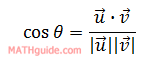The Angle Between Two Vectors
View the Lesson | MATHguide homepage Updated July 17th, 2022

 Problem: Find the angle between these two vectors in degreesto the nearest tenth. u = < 7, -18 >, v = < -13, 3 > Formula:Solution: °